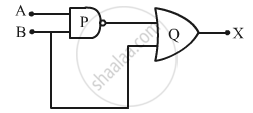# Identify the Logic Gates Marked 'P' and 'Q' in the Given Circuit. Write the Truth Table for the Combination. - Physics

Identify the logic gates marked 'P' and 'Q' in the given circuit. Write the truth table for the combination.#### Solution

P - NAND GATE
Q - OR GATE

Assume:

X'=bar(A.B)

 A B X'=bar(A.B) X 0 0 1 1 0 1 1 1 1 0 1 1 1 1 0 1
Concept: Digital Electronics and Logic Gates
Is there an error in this question or solution?